# نتائج البحث (10)

View
Selected filters:
• Textbook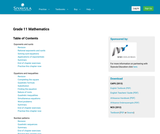Rating

This is a comprehensive math textbook for Grade 11. It can be downloaded, read on-line on a mobile phone, computer or iPad. Every chapter has links to on-line video lessons and explanations. Summary presentations at the end of each chapter offer an overview of the content covered, with key points highlighted for easy revision. Topics covered are: language of mathematics, exponents, surds, error margins, quadratic sequences, finance, quadratic equations, quadratic inequalities, simultaneous equations, mathematical models, quadratic functions and graphs, hyperbolic functions and graphs, exponential functions and graphs, gradient at point, linear programming, geometry, trigonometry, statistics, independent variables, dependent events. This book is based upon the original Free High School Science Text series.

الموضوع:
الرياضيات
نوع المادة:
Textbook
Provider:
موقع Siyavula
Date Added:
04/12/2012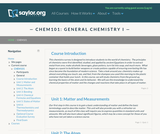Conditions of Use:
قراءة النص المطبوع بخط رفيع
Rating

This survey chemistry course is designed to introduce students to the world of chemistry. In this course, we will study chemistry from the ground up, learning the basics of the atom and its behavior. We will apply this knowledge to understand the chemical properties of matter and the changes and reactions that take place in all types of matter. Upon successful completion of this course, students will be able to: Define the general term 'chemistry.' Distinguish between the physical and chemical properties of matter. Distinguish between mixtures and pure substances. Describe the arrangement of the periodic table. Perform mathematical operations involving significant figures. Convert measurements into scientific notation. Explain the law of conservation of mass, the law of definite composition, and the law of multiple proportions. Summarize the essential points of Dalton's atomic theory. Define the term 'atom.' Describe electron configurations. Draw Lewis structures for molecules. Name ionic and covalent compounds using the rules for nomenclature of inorganic compounds. Explain the relationship between enthalpy change and a reaction's tendency to occur. (Chemistry 101; See also: Biology 105. Mechanical Engineering 004)

الموضوع:
الكيمياء
نوع المادة:
التقييم
المقرر التعليمي الكامل
Homework/Assignment
Lecture
ملاحظات المحاضرة
القراءة
Syllabus
Textbook
Provider:
The Saylor Foundation
Date Added:
11/16/2011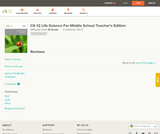Conditions of Use:
يمكن إعادة ترتيب المحتوى ومشاركته
Rating

تقوم جوائز علوم الحياة للمدارس الإعدادية والمقدمة من مؤسسة CK-12 بتغطية سبع وحدات: فهم الكائنات الحية؛ الخلايا: مادة بناء الحياة؛ علم الوراثة والتطور؛ بدائيات النوى والطلائعيات والفطريات والنباتات؛ المملكة الحيوانية؛ جسم الإنسان؛ علم البيئة.

الموضوع:
علم الأحياء
نوع المادة:
Activity/Lab
Textbook
Provider:
CK-12 Foundation
Provider Set:
كتاب FlexBook من تصنيف المرحلة من الابتدائية إلى الثانوية
المؤلف:
Wilkin, Douglas (Editor)
Date Added:
04/21/2010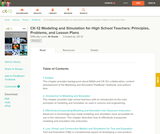Conditions of Use:
يمكن إعادة ترتيب المحتوى ومشاركته
Rating

A collaboration between the National Aeronautics and Space Administration (NASA) and the CK-12 Foundation, this book provides high school mathematics and physics teachers with an introduction to the main principles of modeling and simulation used in science and engineering. An appendix of lesson plans is included.

الموضوع:
الهندسة
الفيزياء
نوع المادة:
Lesson Plan
Teaching/Learning Strategy
Textbook
Provider:
CK-12 Foundation
Provider Set:
كتاب FlexBook من تصنيف المرحلة من الابتدائية إلى الثانوية
Date Added:
10/24/2012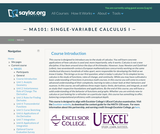Conditions of Use:
قراءة النص المطبوع بخط رفيع
Rating

This course is designed to introduce the student to the study of Calculus through concrete applications. Upon successful completion of this course, students will be able to: Define and identify functions; Define and identify the domain, range, and graph of a function; Define and identify one-to-one, onto, and linear functions; Analyze and graph transformations of functions, such as shifts and dilations, and compositions of functions; Characterize, compute, and graph inverse functions; Graph and describe exponential and logarithmic functions; Define and calculate limits and one-sided limits; Identify vertical asymptotes; Define continuity and determine whether a function is continuous; State and apply the Intermediate Value Theorem; State the Squeeze Theorem and use it to calculate limits; Calculate limits at infinity and identify horizontal asymptotes; Calculate limits of rational and radical functions; State the epsilon-delta definition of a limit and use it in simple situations to show a limit exists; Draw a diagram to explain the tangent-line problem; State several different versions of the limit definition of the derivative, and use multiple notations for the derivative; Understand the derivative as a rate of change, and give some examples of its application, such as velocity; Calculate simple derivatives using the limit definition; Use the power, product, quotient, and chain rules to calculate derivatives; Use implicit differentiation to find derivatives; Find derivatives of inverse functions; Find derivatives of trigonometric, exponential, logarithmic, and inverse trigonometric functions; Solve problems involving rectilinear motion using derivatives; Solve problems involving related rates; Define local and absolute extrema; Use critical points to find local extrema; Use the first and second derivative tests to find intervals of increase and decrease and to find information about concavity and inflection points; Sketch functions using information from the first and second derivative tests; Use the first and second derivative tests to solve optimization (maximum/minimum value) problems; State and apply Rolle's Theorem and the Mean Value Theorem; Explain the meaning of linear approximations and differentials with a sketch; Use linear approximation to solve problems in applications; State and apply L'Hopital's Rule for indeterminate forms; Explain Newton's method using an illustration; Execute several steps of Newton's method and use it to approximate solutions to a root-finding problem; Define antiderivatives and the indefinite integral; State the properties of the indefinite integral; Relate the definite integral to the initial value problem and the area problem; Set up and calculate a Riemann sum; Estimate the area under a curve numerically using the Midpoint Rule; State the Fundamental Theorem of Calculus and use it to calculate definite integrals; State and apply basic properties of the definite integral; Use substitution to compute definite integrals. (Mathematics 101; See also: Biology 103, Chemistry 003, Computer Science 103, Economics 103, Mechanical Engineering 001)

الموضوع:
علم الحساب
نوع المادة:
التقييم
المقرر التعليمي الكامل
Homework/Assignment
القراءة
Syllabus
Textbook
Provider:
The Saylor Foundation
Date Added:
11/11/2011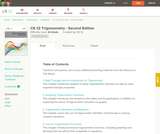Conditions of Use:
يمكن إعادة ترتيب المحتوى ومشاركته
Rating

CK-12 Foundation's Trigonometry FlexBook is an introduction to trigonometry for the high school student. Topics include: Trigonometric Identities & Equations, Circular Functions, and Polar Equations & Complex Numbers.

الموضوع:
علم المثلثات
نوع المادة:
Textbook
Provider:
CK-12 Foundation
Provider Set:
كتاب FlexBook من تصنيف المرحلة من الابتدائية إلى الثانوية
المؤلف:
Fortgang, Art
Hayes, Andrea
Jordan, Lori
Landers, Mara
Meery, Brenda
Ottman, Larry
Date Added:
08/12/2010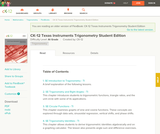Conditions of Use:
يمكن إعادة ترتيب المحتوى ومشاركته
Rating

CK-12's Texas Instruments Trigonometry Student Edition Flexbook is a helpful companion to a trigonometry course, providing students with more ways to understand basic trigonometric concepts through supplementary exercises and explanations.

الموضوع:
علم المثلثات
نوع المادة:
Textbook
Provider:
CK-12 Foundation
Provider Set:
كتاب FlexBook من تصنيف المرحلة من الابتدائية إلى الثانوية
المؤلف:
Jordan, Lori
Date Added:
12/14/2010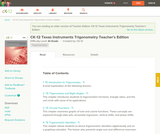Conditions of Use:
يمكن إعادة ترتيب المحتوى ومشاركته
Rating

CK-12's Texas Instruments Trigonometry Teacher's Edition Flexbook is a helpful companion to a trigonometry course, providing students with more ways to understand basic trigonometric concepts through supplementary exercises and explanations.

الموضوع:
علم المثلثات
نوع المادة:
Textbook
Provider:
CK-12 Foundation
Provider Set:
كتاب FlexBook من تصنيف المرحلة من الابتدائية إلى الثانوية
المؤلف:
Jordan, Lori
Date Added:
12/21/2010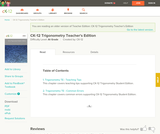Conditions of Use:
يمكن إعادة ترتيب المحتوى ومشاركته
Rating

CK-12 Trigonometry Teacher's Edition provides tips and common errors for teaching CK-12 Trigonometry Student Edition. The solution and assessment guides are available upon request.

الموضوع:
علم المثلثات
نوع المادة:
Textbook
Provider:
CK-12 Foundation
Provider Set:
كتاب FlexBook من تصنيف المرحلة من الابتدائية إلى الثانوية
المؤلف:
Rawley, Eve
Date Added:
06/25/2011Conditions of Use:
بلا تقييدات
Rating

Writing Commons aspires to be a community for writers, a creative learning space for students in courses that require college-level writing, a creative, interactive space for teachers to share resources and pedagogy. Our primary goal is to provide the resources and community students need to improve their writing, particularly students enrolled in courses that require college-level writing. As mentioned in 'About Us', we believe learning materials should be free for all students and teachers part of the cultural commons. Hence, we provide free access to an award-winning, college textbook that was published by a major publisher and awarded the Distinguished Book Award by Computers and Composition: an International Journal.

الموضوع:
English Language Arts
نوع المادة:
القراءة
Textbook
Provider:
المشاع الكتابي
Date Added:
03/30/2012